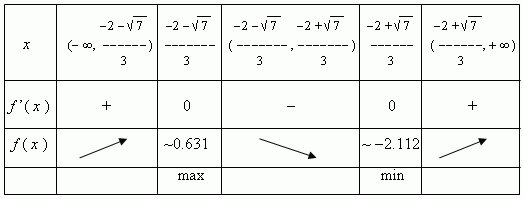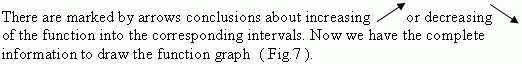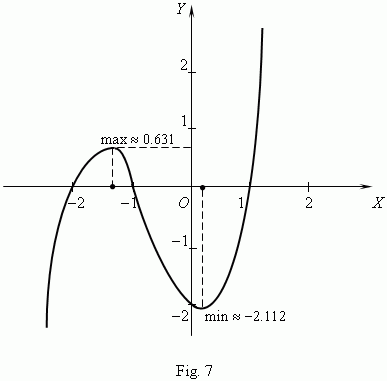# Application of derivative in investigation of functions

Continuity and differentiability of function. Sufficient
conditions of functions monotony. Darboux's theorem.
Intervals of function monotony. Critical points.
Extreme ( minimum, maximum ). Points of extreme.
Necessary condition of extreme. Sufficient conditions
of extreme. Plan of function investigation.

Relation between continuity and differentiability of function. If a function is differentiable at some point, then it is a continuous function at this point. Contrary is invalid: a continuous function can have no derivative.
C o n s e q u e n c e . If a function is discontinuous at some point, then it has no derivative in this point.

 E x a m p l e . The function y = | x |  ( Fig.3 )  is continuous everywhere, but it has no derivative at x = 0 , because a tangent of the graph at this point does not exist.  ( think, please, why ? )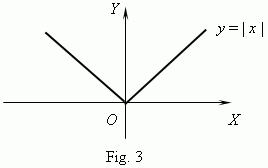Sufficient conditions of functions monotony.
If   f ’( x ) > 0  at every point of an interval ( a, b ) , then a function   f ( x ) increases within this interval.
If   f ’( x ) < 0  at every point of an interval ( a, b ) , then a function   f ( x ) decreases within this interval.

Darboux’s theorem. Points, at which a derivative of a function is equal to 0 or doesn’t exist, divide a function domain for such intervals that within each of them a derivative saves a constant sign.

Using these signs it is possible to find intervals of monotony of functions, what is very important in investigations of functions.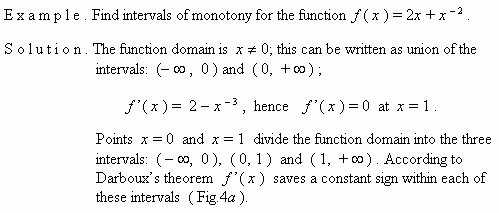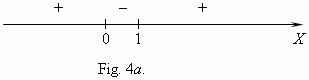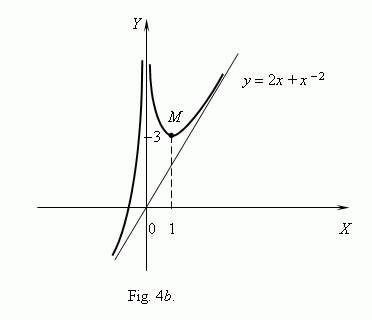Hence, the function increases in the intervals ( -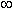, 0 ) and ( 1, +) and
decreases in the interval ( 0,  1 ). The point x = 0  isn’t included in the function
domain, but as x approaches 0 an item x - 2 increases unboundedly, therefore
the function also increases unboundedly. At the point x = 1  the function value
is 3. According to this analysis  we can draw the graph of the function,
represented on  Fig.4 b .

Critical points. A domain interior points, in which a derivative of a function is equal to zero or doesn’t exist, are called critical points of this function. These points are very important at analysis and drawing a function graph, because only they can be points, in which a function has an extreme ( minimum or maximum ,  Fig.5 a, b ).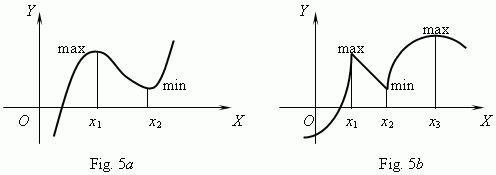At points x 1 , x 2 ( Fig.5 a ) and x 3 ( Fig.5 b ) a derivative is equal to 0; at points x 1 , x 2 ( Fig.5 b ) a derivative doesn’t exist. But all they are points of extreme.

Necessary condition of extreme. If   x 0 is an extreme point of a function  f ( x ) and a derivative  f’  exists at this point, then  f’ ( x 0 ) = 0 .

This theorem is a necessary condition of extreme. If a derivative of a function at some point is equal to zero, then it’s not necessarily, that the function has an extreme at this point. For instance, a derivative of the function f ( x ) = x ³  is equal to 0  at x = 0 , but this function has no extreme at this point  ( Fig.6 ).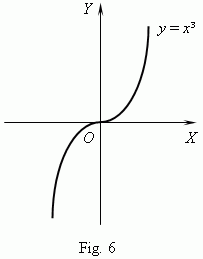On the other hand, the function y = | x | , represented on Fig.3, has a minimum at the point x = 0 , but there is no derivative at this point.

Sufficient conditions of extreme. If a derivative changes its sign from plus to minus at a point  x 0 , then  x 0 is a point of maximum.  If a derivative changes its sign from minus to plus at a point  x 0 , then  x 0 is a point of minimum.

Plan of function investigation. To draw a graph of a function it is necessary:

1)  to find a domain and a codomain of a function,

2)  to ascertain if the function is even or odd,

3)  to determine if the function is periodic or not,

4)  to find zeros of the function and its values at x = 0,

5)  to find intervals of a sign constancy,

6)  to find intervals of monotony,

7)  to find points of extreme and values of a function in these points,

8)  to analyze the behavior of a function near “special” points and at a great modulus of x .

E x a m p l e . Analyze the function f ( x ) = x ³ + 2 x ² - x - 2 and draw its graph.

S o l u t i o n . We’ll investigate the function by the above represented scheme.

1)  a domain x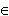R ( i.e. x – any real number ); a codomain yR , because
f ( x )  is an odd degree polynomial;
2) f ( x ) is neither an even nor an odd function ( explain, please );
3) f ( x ) is not a periodic function ( prove this, please, yourself );
4)  a graph of this function is intersected with y -axis in the point ( 0, - 2 ),
because f ( 0 ) = - 2 ;  to find zeros of the function it is necessary to solve
the equation: x ³ + 2 x ² - x - 2  = 0 , one of roots of which ( x = 1 ) is
obvious. Other roots can be found ( if they exist ! ) by solving the quadratic
equation: x ² + 3 x + 2 = 0, which is received after dividing the polynomial
x ³ + 2 x ² - x - 2  by the binomial  ( x – 1 ). It is easy to check, that the
two other roots are: x 2 = - 2 and x 3 = - 1. So, zeros of this function are:
- 2, - 1 and 1.
5)  It means, that a numerical line is divided by these roots into four intervals of
a sign constancy, inside of which the function saves
its sign :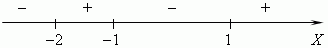This result can be received from the polynomial factorization:

x ³ + 2 x ² - x - 2 = ( x + 2 ) ( x + 1 ( x – 1 )

and estimating the product sign by the method of intervals .
6)  The derivative: f’ ( x ) = 3 x ² + 4 x - 1  has no points, at which it doesn’t
exist, therefore its domain is R ( all real numbers ); zeros of f’ ( x ) are
roots of the equation:  3 x ² + 4 x - 1 = 0 .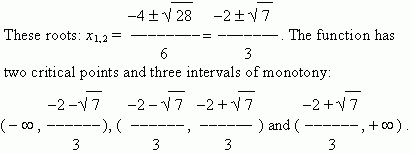The received results are included in the following table: